# Diffusion-Based Molecular Communication in Next Generation Networks

Diffusion-Based Molecular Communication in Next Generation Networks
Department of Electrical Engineering Indian Institute of Technology, Jammu 181121, India

Corresponding Author Email:
yusrabanday23@gmail.com
Page:
9-14
|
DOI:
https://doi.org/10.18280/ama_b.651-402
14 September 2022
| |
Accepted:
5 November 2022
| | Citation

OPEN ACCESS

Abstract:

With the advancement of bioengineering and nano-technology, next-generation network architecture is being equipped with nano-devices to improve its scalability. Inspired by naturally existing biological phenomena of communication, molecular communication-based nano-networks are designed on the same principles. In existing communication systems, information is transmitted by electromagnetic or electrical signals. However, these methods of communication are inconvenient for many applications where the ratio of antenna size to the wavelength of a signal is a constraint. In such scenarios, a molecular communication scheme can be employed to solve such issues. Here chemical signals act as information carriers. These signals are biocompatible and can be used in body area networks (BANs). In this paper, a nano-network in which communication through diffusion takes place is simulated and evaluated for various signal metrics (delay, distortion, bit rate). The concentration of molecules in pheromone signaling communication can be used as a channel transfer function in the respective molecular communication model used in nano-devices. A stochastic Single-Input-Single-Output (SISO) communication system is simulated for purpose of analysis.

Keywords:

molecular communication, 5G, stochastic model, SISO

1. Introduction

Traditional electromagnetic waves cannot be used for intra-body sensor communication in Body Area Networks (BANS). The main reason behind this fact is that classical Radio Frequency (RF) and electromagnetic signals are non-biocompatible for use inside the body because of their high energy consumption and electromagnetic interference . To overcome this issue, one possible solution is the use of chemical signals instead of RF. Chemical signaling plays a vital role to connect the life processes of both humans and microorganisms. These signals are used to communicate and transmit information from sender to receiver cells. Here also, a communication system is similar to the one used for the propagation of information in form of analog and digital signals in the RF domain. The transmitter releases small molecules in coded form as communication carriers in a channel which is usually in gaseous or liquid form. These coded molecules propagate through a medium (channel) and reach the receiver. A receiver decodes these molecules and extracts information from them. This form of communication aided by molecules as information carriers is called Molecular Communication. Based on the mode of propagation there are three types of molecular communication viz; diffusion-based, walkway-based, and flow-based . In this work, we consider an environment where molecules propagate through a process of diffusion. Diffusion is a process of flow of molecules from the area of their higher concentration to the lower concentration.

Transmitters and receivers used here are nano-machines created artificially and biologically. These are designed to emit and capture molecules. Information-carrying molecules are hormones or neurotransmitters. Information molecules are in the form of ions, proteins, or DNA. It makes communication possible between nano-machines with the help of nano-sized particles. Molecular communication mimics nature where communication is usually done through molecules and chemical signals. Inter-cellular and intra-cellular communication is done through neurotransmitters, and vesicle transport at micro and nano-scales respectively . These key features not only make molecular communications biocompatible but also result in low consumption of energy required for the generation and propagation of chemical signals. Owing to the use of biological properties of cells and molecules, it facilitates communication in an aqueous medium. The traditional communication techniques lack this feature  and hence cannot be used for intra-organ communication within the body.

This work is motivated by the fact that conventional radio frequency technologies are incompatible with applications in body area networks (BANs). Thus DMC emerges as a compatible system in body environments. Nano-technology allows nanomachines to provide an interface between electronic and biological systems. To address the increase in the number of heart diseases, usually, a pacemaker is considered to be a possible solution. To ensure improved performance over lead-based pacemakers, a study is carried out on leadless pacemakers. Therefore, intra-body nanonetworks are envisaged to be combined with DMC and implemented in the human body. The communication of these nanomachines is still a challenging phenomenon. In this work, we have investigated and analyzed the functioning and performance of leadless pacemakers in DMC.

In this paper, we have analyzed and envisioned the use and performance of nano-devices within the human body. The mortality rate due to cardiac failures is increasing day by day. So there arises a need for technology to help in the early detection of such diseases and save the lives of patients. Thus the high volume of data involved needs a huge bandwidth, and a reliable system. Therefore 5G provides the low-latency, ultra-reliable framework for such diffusion-based molecular communications. In congestive heart failure (CHF), muscles and arteries of the heart become impaired and make it difficult to pump blood according to the needs of the human body . In such conditions when the heart beats too rapidly or slowly due to abnormal heart rhythms, the required amount of blood does not reach the body. This causes shortness of breath and fatigue. To treat this condition a small device called a pacemaker is used to bring the heart rate back to normal . Traditional pacemakers that use lead cause many complications such as lead fracture, insulation failure, and skin erosions . This necessitates the use of leadless or wireless pacemakers which can be placed in the heart directly . These pacemakers comprise Transmitter, Channel, and Receiver. The performance of these nano-devices is evaluated by estimating the metrics like Inter symbol Interference (ISI), distortion, gain, and data rate. In this paper, an investigation is done to study how diffusion-based molecular communication improves the efficiency of a pacemaker for the heart.

In recent research related to the development of nanomachines, several models have been proposed. In reference , a naturally existing MC mechanism, the information signals are encoded by the transmitter and transmitted to a receiver. In this work, we have used diffusion-based MC. In reference , a study has been carried out with the environment as blood vessels and one blood flow in one dimension. This study considers the heart as a medium and the flow of molecules in all three dimensions.

For simplification of simulations and mathematical analysis, a communication system is represented in one dimension.

The rest of the paper is organized as follows. Section 2 gives a system model of a point-to-point deterministic SISO diffusion-based molecular communication network modeled between leadless pacemakers. In section 3, an approach to evaluate the performance of the concentration model is explained. Various parameters used for performance analysis are also described. In section 4, an elaborated deterministic SISO model of the physical diffusion channel is presented. Simulation results are explained in section 5, and the paper is concluded in section 6.

2. System Model

The block diagram of a molecular communication system with SISO communication as shown in Figure 1 consists of the encoder, the channel, and the decoder.

## 1.png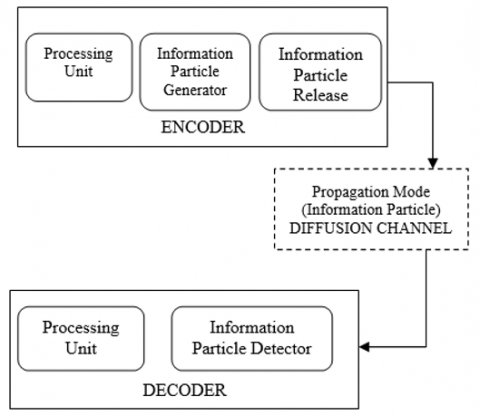Figure 1. Block diagram of molecular communication

The encoder uses amplitude modulation, and on-off keying (OOK) . In this form of amplitude modulation, molecules are sent if the input signal is present, i.e., $X i=1$.

If molecules are not sent, then the input signal is $X i=0$.

2.2 The propagation channel

In diffusion-based molecular communications, the physical channel is different from the one used in traditional communication systems . This propagation of molecules in this channel follows the Brownian motion model in which molecules move randomly at a different speed and time. This causes a crossover effect in which different molecules arrive in a time interval different than intended . Due to this, it cannot be used in the human body, especially in cardiac systems where the timing of signals plays a vital role in the proper functioning of the heart.

A binary hypothesis testing channel is widely used for optimal detection in such cases. This channel uses two hypotheses, H0and H1 where;

$\left\{\begin{array}{l}H_0: \text { Nomolecules sent } \\ H_1: \text { Molecules are sent }\end{array}\right.$     (1)

This channel has four different probabilities; the probability of detection, $P_D$, gives the probability of receiving all the molecules that are transmitted. The second one is the probability of false alarm $P_{\mathrm{F}}$. This gives the probability of molecules sent, but not received. The remaining two probabilities are the inverses of these two as shown in Figure 2 .

## 2.pngFigure 2. Binary hypothesis testing channel

Thus from the above definition, we can present the binary channel mathematically as:

$\begin{gathered}\left(Y_i=1 \mid X_i=0\right)=P_F \\ \left(Y_i=1 \mid X_i=1\right)=P_D \\ \left(Y_i=0 \mid X_i=0\right)=1-P_F \\ \left(Y_i=0 \mid X_i=1\right)=1-p_D\end{gathered}$    (2)

2.3 The decoder

The blood in the heart flows in three dimensions. In such cases to get an optimal decision threshold for inputs 0 and 1, a priori information should be known at the receiver. The decoder used for this purpose is a Neyman-Pearson detector .

In this section Concentration Model of the Heart is explained in which the channel of a communication system is based on molecular communications. To simplify the mathematical analysis, the molecular communication concentration models for blood flow used earlier were based on the cardiovascular system instead of the heart. This is because the velocity of blood flow in vessels is unidirectional. While as in the heart the blood flow velocity is in three dimensions. This makes performance analysis complex .

The model of a diffusion-based communication system in plants using pheromones as information particles is discussed in reference . To evaluate the performance of this model, the concentration of the molecules is analyzed and calculated as :

$(\vec{x},t)=\frac{{{Q}_{T}}}{8{{\left( \pi r \right)}^{{}^{3}/{}_{2}}}}{{e}^{\left( -{{\left( x-ut \right)}^{2}}-{{y}^{2}} \right)/4r}}\left[ {{e}^{-{{\left( z-H \right)}^{2}}/4r}}+{{e}^{-{{\left( z+H \right)}^{2}}/4r}} \right]$      (3)

$c(\vec{x}, t)$ gives a concentration profile of pheromones at position $\vec{x}=(x, y, z)$ at time $t$, emitted instantaneously from the transmitter plant. This gives the impulse response in three dimensions.

In reference , $r$ is redefined as a new variable such that $r=\frac{K x}{u} \mathrm{~m}^2$.

$\therefore (\vec{x},t)=\frac{{{Q}_{T}}}{8{{\left( \frac{\pi Kx}{u} \right)}^{{}^{3}/{}_{2}}}}{{e}^{\left( -{{\left( x-ut \right)}^{2}}-{{y}^{2}} \right)/\frac{4Kx}{u}}}\left[ {{e}^{-{{\left( z-H \right)}^{2}}/\frac{4Kx}{u}}}+{{e}^{-{{\left( z+H \right)}^{2}}/\frac{4Kx}{u}}} \right]$     (4)

Table 1. Comparison of the parameters used in the pheromone and molecular communications

 Parameters Definition in pheromone communications in wind Definition in molecular communications in the heart $c(\vec{x}, t)$ Concentration of pheromones Concentration of molecules $\vec{x}=(x, y, z)$ The position of pheromones The position of the molecules $u$ The wind velocity Blood flow velocity K The diffusion coefficient represents the turbulent eddy diffusivities in (x; y; z) directions Eddy diffusivities in (x; y; z) directions H Height of the transmitting leaf from the ground The closest distance from the pacemaker to the wall of the heart QT The number of pheromones released from the plants per second The number of molecules released from the transmitter pacemaker per second

Both the molecular and pheromone communications transfer information from source to destination through a process of diffusion, hence same equations are used to calculate the concentration of molecules. However, in this paper, all the parameters used to calculate the concentration of molecules in the heart are redefined as shown in comparison Table 1.

For a similar use of parameter H in both cases, some assumptions are made as under:

-The molecules reach the closest wall only.

-Blood flow velocity is constant.

-Nano-device system includes one transmitter pacemaker, $T x$, and one receiver pacemaker, $R x$.

-Both pacemakers are placed at equal distances from their respective closest walls of the heart as shown in Figure 3. Red dots in the figure represent information molecules transmitted by transmitter sensor Tx and received at receiver sensor $R x$.

## 3.png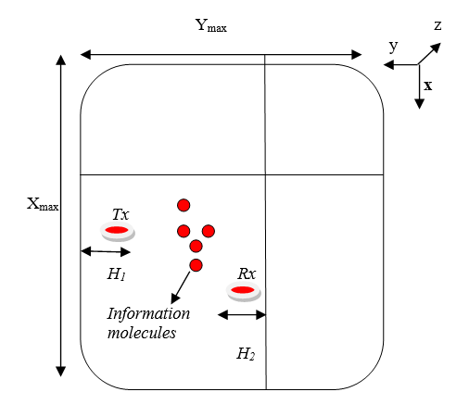Figure 3. Model of heart in 3D with leadless pacemakers

These molecules travel randomly in the propagation channel towards destination $R x$. Axes $\mathrm{x}, \mathrm{y}$, and $\mathrm{z}$ give a $3-\mathrm{D}$ presentation of the heart. $\mathrm{X}$ gives the height, $\mathrm{y}$ is the width and $\mathrm{z}$ is the depth of the heart. From the last assumption $H_1=H_2=$ $H$.

3. Performance Analysis of the Deterministic SISO Concentration Model

To carry out the performance analysis of the model shown in Figure 3, values of all the parameters given in Table 1 are to be calculated. The velocity of the blood flow is measured to be approximately 15 cm/s . The value of the eddy diffusivities in 3-D is given by :

K=RD      (5)

where,

R=Reynolds number

D=diffusion coefficient of molecules=$e^{-6} \mathrm{~m}^2 / \mathrm{s}$ 

The Reynolds number is defined as :

$R=\frac{u L}{v}$       (6)

where,

u=blood flow velocity

L=diameter of the heart

$v=\frac{\mu}{\rho}=$ the blood kinematic viscosity      (7)

\begin{aligned} & \mu=\text { blood viscosity }=(3 \sim 4)^{-3} \mathrm{~Pa} \text {-seconds } \\ & \rho=\text { blood density }=1.06 e^3 \mathrm{~g} / \mathrm{m}^3[15,17]\end{aligned}

Assuming the heart to be the size of a closed fist, the value of $\mathrm{H}$ is taken to be $7.5 \mathrm{~mm}$. The number of molecules transmitted $Q_T$ is assumed to be 500 . Now the simulations are carried out to evaluate the performance of the model.

Figure 4 can be further simplified and modeled as a cylinder with $T x$ and $R x$ pacemakers positioned along a vertical axis.

## 4.png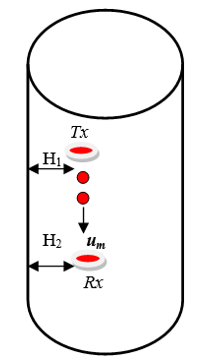Figure 4. Simplified heart model

4. Results and Discussion

On simulating the concentration of the molecules c (x,y,z,t), a contour plot is obtained as shown in Figure 5.

## 5.png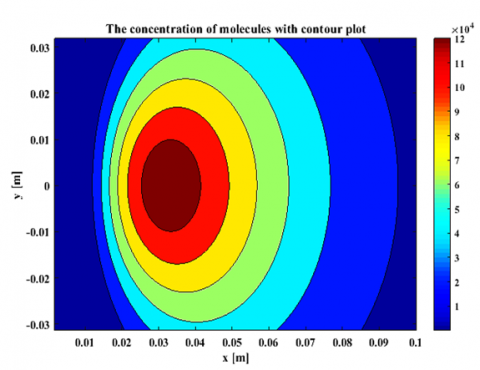Figure 5. The concentration of the molecules depends on the distance traveled in the x- and y-direction

The figure shows that the concentration of molecules diffuses from the Tx pacemaker toward the Rx pacemaker at an increased rate. The inner maroon color circle is a point of emission of molecules from Tx. Here red, yellow, green, and blue circles represent the diffusion of information molecules to  Rx.

In traditional communication systems, some parameters that are used to explain the performance are gain, delay, distortion, and attenuation. In diffusion-based molecular communication same parameters are used for performance analysis.

Attenuation or gain in molecular communication is defined as a ratio of maximum concentration Ct and absolute maximum value of concentration Co at any time instant.

$gain=\frac{{{c}_{t}}}{{{c}_{o}}}=\frac{\text{max }\!\!\{\!\!\text{ c(x,y,z,t) }\!\!\}\!\!\text{ }}{\max [\max \{c(x,y,z,t)\}]}$      (8)

Delay is defined as the total time it takes for a molecule to travel a distance between Tx and Rx until the concentration at the receiver becomes maximum.

$delay\text{ }=\text{ }\arg \max \{c(x,y,z,t)\}$     (9)

From Eqns. (8) and (9) a concentration versus time plot is obtained as in Figure 6.

## 6.png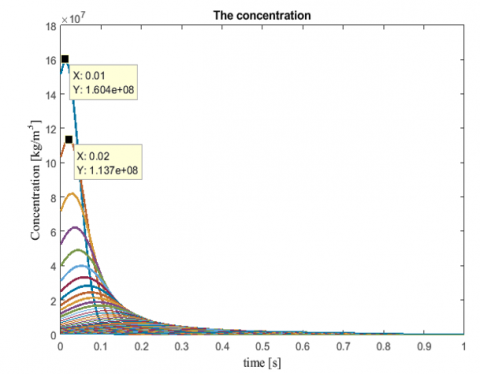Figure 6. The concentration of the molecules Vs  the distance in the x-direction

The curves in Figure 6 show the concentration of the molecules at different distances from the transmitter at different time instants. The peak value of $1.604 \times 10^8 \mathrm{~kg} / \mathrm{m} 3$ represents an absolute maximum value of the concentration, $c_0$ at approximately $0.01 \mathrm{~s}$. This curve with the highest concentration represents the closest distance to the transmitter. The curve of the lowest concentration represents the distance farthest from the transmitter. For different values of time and distance from the transmitter different curves are obtained. This causes variation in delay for different concentration curves. With an increase in the distance, the delay of the maximum concentration also increases. This is due to the molecules diffusing away from the area of their maximum concentration (Tx) towards the area of their minimum concentration (Rx). From figure 6 it can be also seen that the maximum concentration gradually decreases in magnitude with the flow of molecules till it is evenly distributed in the channel.

Gain and delay graphs are separately plotted with respect to the distance from the transmitter as shown in Figures 7 and 8 respectively. These plots show how gain and delay vary according to the distance the molecule travels.

The gain in the above graph is plotted on a logarithmic scale to analyze all the small variations. At the distance closest to the transmitter, the ratio between the maximum and the absolute maximum concentration is unity hence the gain is 0 dB. As distance is increased, the gain decreases. It can be observed that the maximum concentration decreases relative to the absolute maximum concentration as the molecules move toward the receiver.

## 7.png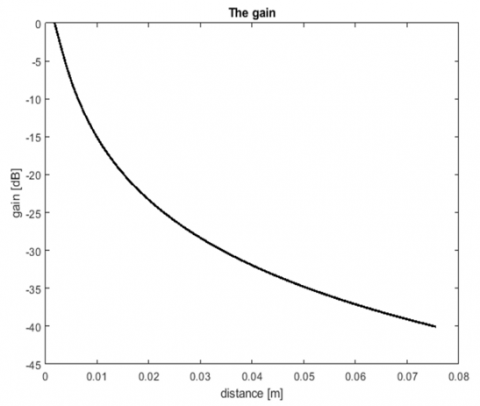Figure 7. The gain of concentration

## 8.png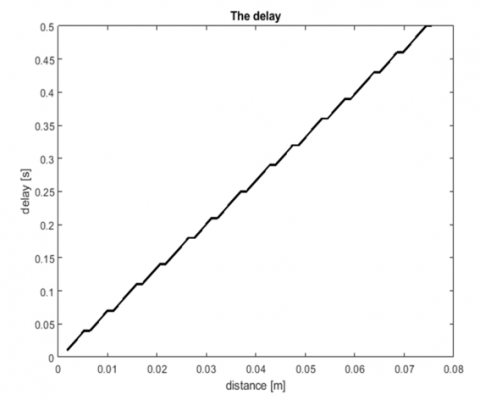Figure 8. The delay in the concentration

From Figure 8, the delay increases slowly with the distance from the transmitter. For certain time intervals, the delay is constant for some distance. This is probably due to the time resolution in the simulations. If the time interval is reduced these constant delays are eliminated. This delay graph shows that the concentration model is a perfect model for the communication system.

5. Conclusion

The concept of diffusion-based molecular communications used in leadless pacemakers is introduced. A Single-Input-Single-Output (SISO) communication model with deterministic signaling is explained. An on-off keying (OOK) encoder is used. The diffusion channel is modeled by using a binary hypothesis-testing channel. A Neyman Pearson detector, based on the maximum likelihood test is used for decoding the information.

Since the communication system is based on molecular diffusion, the concentration model of the molecules is studied in detail. The mathematical model for pheromone communications is mapped to the concentration of the molecules in the diffusion-based molecular communication system.

The plots obtained from the performance analysis of the gain and delay signify that the concentration model anticipates the diffusion from the transmitter to the receiver pacemaker. The maximum peak of concentration is obtained when the transmitter just transmits the molecules. The concentration decreases when the time and distance between the transmitter and receiver increase. Also, as the signaling intervals increase, the data rate increase, and distortion decreases. The SISO communication system should be used when high capacity is required. In future work, the blood viscosity can be considered to be a real-time variable instead of using a fixed value as done in this study.

Acknowledgments

This work is supported in part by the Science and Engineering Research Board (SERB) DST, Government of India, under Grant, File No. PDF/2021/002533.

References

 Seyedi, M., Kibret, B., Lai, D.T., Faulkner, M. (2013). A survey on Intrabody communications for body area network applications. IEEE Transactions on Biomedical Engineering, 60(8): 2067-2079. http://dx.doi.org/10.1109/TBME.2013.2254714

 Fereidouni, A. (2013). Analysis of connectivity in diffusion-based molecular nano communication networks. Doctoral dissertation, Eastern Mediterranean University, Turkey.

 Johnson, A., Lewis, J., Raff, M., Roberts, K., Walter, P. (2002). Molecular biology of the cell. Garland Science. http://dx.doi.org/10.1201/9781315735368

 Hiyama, S., Moritani, Y., Suda, T. (2008). Molecular transport system in molecular communication. NTT DOCOMO Technical Journal, 10(3): 49-53.

 Congestive Heart Failure and Heart Disease. https://www.webmd.com/heart-disease/guide-heart-failure accessed on Jun. 17, 2022.

 Marqués-Gálvez, J.E., Veneault-Fourrey, C., Kohler, A. (2022). Ectomycorrhizal symbiosis: From genomics to trans-kingdom molecular communication and signaling. In Microbial Cross-talk in the Rhizosphere, pp. 273-296. http://dx.doi.org/10.1007/978-981-16-9507-0_11

 Garg, J., Reddy, V.Y. (2019). Leadless cardiac pacemakers: Paradigm shift in cardiac pacing. Heart Rhythm, 16(1): 72-73. https://doi.org/10.1016/j.hrthm.2018.10.012

 Parollo, M., Barletta, V., Mazzocchetti, L., Gentile, F., De Lucia, R., Viani, S., Segreti, L., Di Cori, A., Zucchelli, G., Bongiorni, M.G. (2022). Feasibility and long-term outcomes of leadless pacemaker implant after transvenous lead extraction. Europace, 24(S1), euac053-414. http://dx.doi.org/10.1093/europace/euac053.414

 Suda, T., Moore, M., Nakano, T., Egashira, R., Enomoto, A. (2005). Exploratory research on molecular communication between nanomachines. In Genetic and Evolutionary Computation Conference (GECCO)’05, Washington, DC, USA.

 Felicetti, L., Femminella, M., Reali, G. (2013). Establishing digital molecular communications in blood vessels. In 2013 First International Black Sea Conference on Communications and Networking (BlackSeaCom), Batumi, Georgia, pp. 54-58. http://dx.doi.org/10.1109/BlackSeaCom.2013.6623381

 Yeh, P.C., Chen, K.C., Lee, Y.C., Meng, L.S., Shih, P.J., Ko, P.Y., Lin, W.A., Lee, C.H. (2012). A new frontier of wireless communication theory: Diffusion-based molecular communications. IEEE Wireless Communications, 19(5): 28-35. http://dx.doi.org/10.1109/MWC.2012.6339469

 Unluturk, B.D., Akyildiz, I.F. (2016). An end-to-end model of plant pheromone channel for long-range molecular communication. IEEE transactions on nano Bioscience, 16(1): 11-20. http://dx.doi.org/10.1109/TNB.2016.2628047

 Noe, S.M., Ciccioli, P., Brancaleoni, E., Loreto, F., Niinemets, Ü. (2006). Emissions of monoterpenes linalool and ocimene respond differently to environmental changes due to differences in Physico-chemical characteristics. Atmospheric Environment, 40(25): 4649-4662. http://dx.doi.org/10.1016/j.atmosenv.2006.04.049

 Tortora, G.J., Derrickson, B. (2012). The cardiovascular system: Blood vessels and hemodynamics. Principles of Anatomy and Physiology, pp. 610-635.

 Chahibi, Y., Akyildiz, I.F., Balasingham, I. (2016). Propagation modeling and analysis of molecular motors in molecular communication. IEEE Transactions on Nanobioscience, 15(8): 917-927. http://dx.doi.org/10.1109/TNB.2016.2620439

 http://www.grc.nasa.gov/WWW/BGH/reynolds.html accessed on Jun. 17, 2022.

 Cutnell, J.D., Johnson, K.W. (2020). Physics (V.1), John Willey and Son, INC.